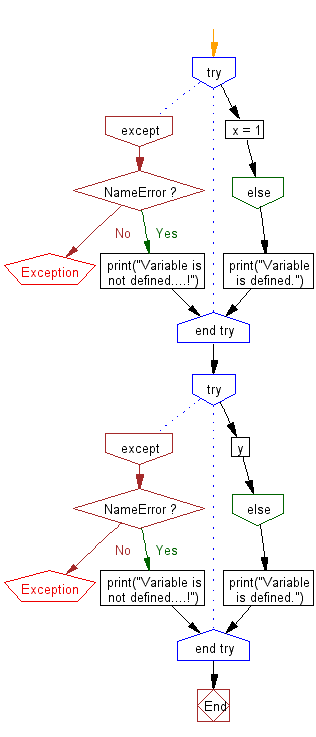﻿ Python: Determine whether variable is defined or not - w3resource# Python: Determine whether variable is defined or not

## Python Basic: Exercise-121 with Solution

Write a Python program to determine whether variable is defined or not.

Sample Solution:

Python Code:

``````try:
x = 1
except NameError:
print("Variable is not defined....!")
else:
print("Variable is defined.")
try:
y
except NameError:
print("Variable is not defined....!")
else:
print("Variable is defined.")
```
```

Sample Output:

```Variable is defined.
Variable is not defined....!
```

Flowchart:## Visualize Python code execution:

The following tool visualize what the computer is doing step-by-step as it executes the said program:

Python Code Editor:

Have another way to solve this solution? Contribute your code (and comments) through Disqus.

What is the difficulty level of this exercise?

Test your Python skills with w3resource's quiz

﻿

## Python: Tips of the Day

Try-catch-else construct:

```try:
foo()
except Exception:
print("Exception occured")
else:
print("Exception didnt occur")
finally:
print("Always gets here")
```